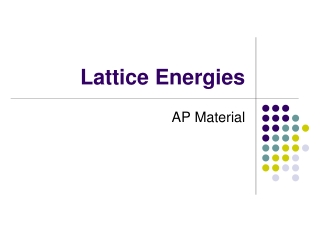DownloadDownload PresentationLattice Energies

# Lattice Energies

Download Presentation## Lattice Energies

- - - - - - - - - - - - - - - - - - - - - - - - - - - E N D - - - - - - - - - - - - - - - - - - - - - - - - - - -
##### Presentation Transcript

1. Lattice Energies AP Material

2. Lattice Energy • Lattice energy: The energy required to completely separate a mole of a solid ionic compound into its elements in their standard states • It is a measure of just how much stabilization results from arranging oppositely charged ions in an ionic solid

3. The principal reason that ionic compounds are stable is the attraction between oppositely charged ions • This attraction draws the ions together, releasing energy and causing ions to form a solid array, or lattice • http://www.youtube.com/watch?v=VBReOjo3ri8&feature=related

4. The formation of ionic compounds is highly exothermic (releasing heat and light): Na(s) + ½ Cl2(g) → NaCl(s) ∆ H = -788kJ/mol • The reverse reaction is highly endothermic: NaCl(s) → Na(s) + ½ Cl2(g) ∆ H = 788kJ/mol

5. Calculating Lattice Energy Eel = кQ1Q2 d where, • К is a constant (8.99 x 109 J-m/C2) • Q1 and Q2 are the charges on the ions • d is the distance between their centers

6. Therefore, the magnitude of the lattice energy is dependant upon: • the charges on the particles (Q1 and Q2) • the distance between the nuclei

7. Summary • For a given arrangement of ions, the lattice energy increases as the charges on the ions increase and as their radii decrease

8. Example: Arrange in order of increasing lattice energy a) NaF, CsI and CaO CsI < NaF < CaO b) ZrO2,MgF2, CaF2 and CaF2 < MgF2 < ZrO2 c) LiF, NaF, CaF2, AlF3 NaF < LiF < CaF2 < AlF3 d) CaCl2, LiCl, Al2O3, NaCl NaCl < LiCl < CaCl2 < Al2O3

9. Lattice energy cannot be determined by experiment It is calculated by envisioning the formation of an ionic compound occurring in steps (Hess’s Law) Called the Born-Haber Cycle after Max Born and Fritz Haber (German scientists) The Born-Haber Cycle

10. Steps in the formation of NaCl 1: Na(s) + ½ Cl2(g) → NaCl(s) ∆Hf = -411kJ 2: Na(s) → Na(g) ∆H°f = 108 kJ 3: 1/2Cl2(g) → Cl(g)∆H°f = 122 kJ 4: Na(g) → Na+(g) + e I1(Na) = 496 kJ 5: Cl(g) + e → Cl-(g) E(Cl) = -396 kJ 6. Overall : ΔH6= ΣΔ H1 to Δ H5